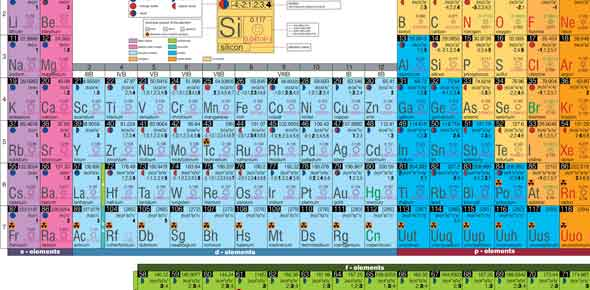# Atoms And The Periodic Table Quiz

10 Questions | Total Attempts: 39SettingsUnderstanding the periodic table gives us a good way of understanding the elements in science. Each element is differentiated by another due to its components and number of items. The quiz below was created to test out a student’s understanding of the atoms and the elements in the periodic table. Why don’t you give it a try and see just how much you recall from your classwork?

• 1.
Which two part of the atom have about the same mass?
• A.

Electrons and shells

• B.

Protons and neutrons

• C.

Orbits and protons

• 2.
The number of protons is the same as the number of electrons which is the same as _____
• A.

The atomic weight

• B.

The atomic number

• C.

The Peroidic family

• 3.
There are  __________ number of elements in the current periodic table
• A.

About 111, but I have not checked Google today to see if a new one was found.

• B.

More than 100 but after that I am a little lost

• C.

Less than 100

• D.

A whole bunch but I am not sure how many, can I go look at the chart?

• 4.
Protons have a  ________________ charge
• A.

Pleasing

• B.

Negative

• C.

Neutral

• D.

Positive

• 5.
The first orbital of an atome can hold
• A.

8 electrons

• B.

2electrons

• C.

6quarks

• D.

4zippos

• 6.
A full outer shell of an atom is called
• A.

A full ring

• B.

A full octet

• C.

A stable octet

• D.

A chubby atom

• 7.
If there are 2 electrons in the first ring and 3 in the second ring, what element are we talking about?  ( run  to the periodic to look real quick)
• A.

Carbon

• B.

Nitrogen

• C.

Boron

• D.

Neon

• 8.
If an atom has 6 electrons in it's outer shell it will want to....
• A.

Give up electrons to become stable

• B.

Gain or take electrons from another element to become stable

• 9.
The term we use when talking about the weight (mass) of an atom is
• A.

An Emu ... energy mass unit

• B.

An Amu ... atomic mass unit

• C.

Watts

• 10.
WHen talking about atomic models is goes in this order
• A.

Dalton's atom model, the gold foil and then plumpudding

• B.

Plum pudding, gold foil and then Dalton's model

• C.

Dalton's atom, Thompson's plum pudding and then Rutherford's gold foil

• D.

Moe, larry, curly

Related TopicsBack to top